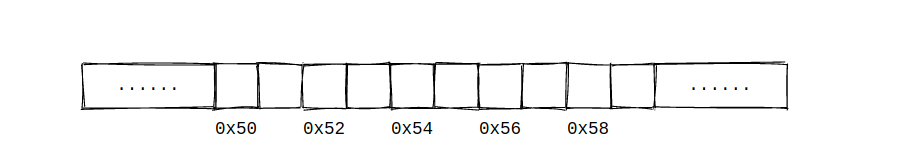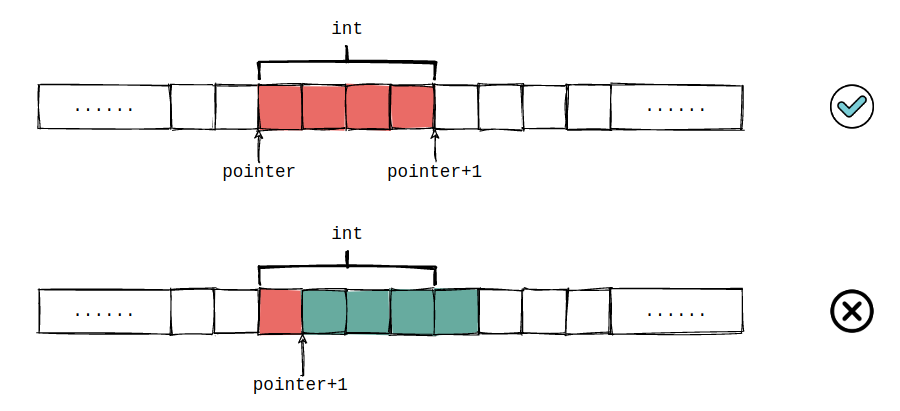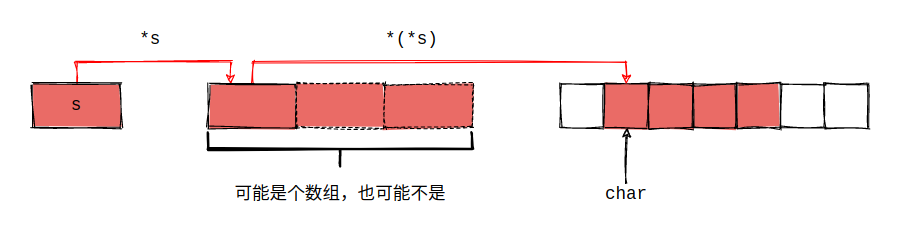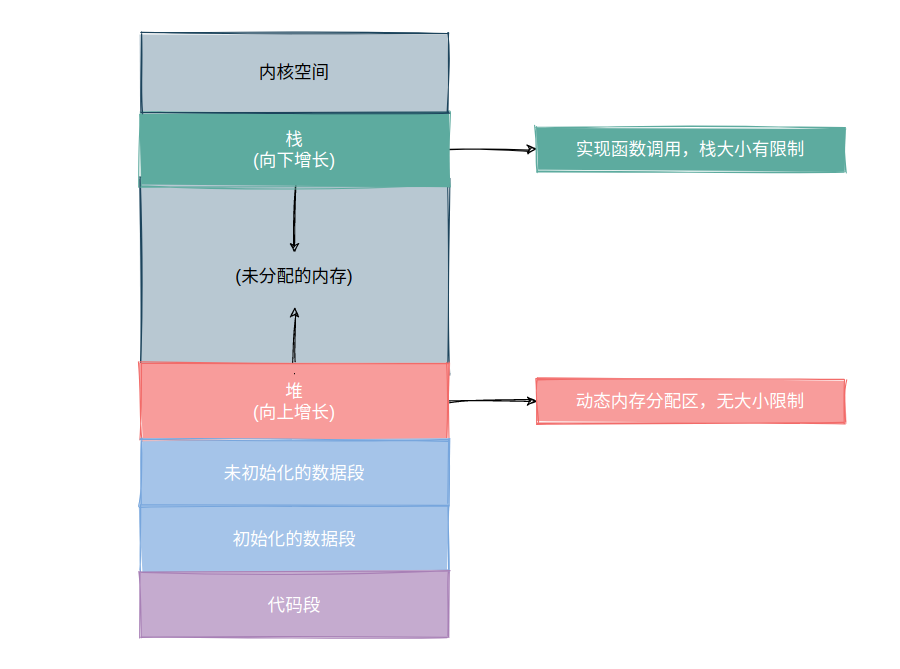C 语言指针真正精髓的地方在于指针可以进行加减法，这一点极大的提升了程序的对指针使用的灵活性，同时也带来了不小的学习负担。正是因为 C 语言指针可运算，才奠定了如今 C 语言的地位。

### 1. 指针

#### 1.1 指针的基本概念``````void main() {
int number;
int *pointer;
}
``````

``````/*
* 所以，当我们将 *pointer 看作是一个整体之后，对于多变量声明就不会再产生疑惑
* *pointer 与 number 的类型均为 int
*/
void main() {
int *pointer, number;
}
``````

#### 1.2 指针的初始化

``````void main() {
int number = 100;       // ①
int *pointer;           // ②

*pointer = number;      // ③
}
``````

``````void main() {
int number = 100;
int *pointer;

pointer = &number;
}
``````

#### 1.3 指针的运算

C 语言中的指针支持运算，不过仅支持加减法，并不支持乘除法。在前面我们已经提到过了，一元运算符 `*` 表示间接寻址，即取出指针的地址，根据该地址找到指针指向的变量。而对于指针的加减法而言，与指针指向的类型密切相关。

``````#include <stdio.h>

void main() {
int *pointer, number = 10;
pointer = &number;

printf("pointer's value is: %p \n", pointer);

pointer = pointer + 1;
printf("pointer's value is: %p \n", pointer);
}

// pointer's value is: 0x7ffd0df7f61c
// pointer's value is: 0x7ffd0df7f620
``````#### 1.4 指针与数组

``````#include <stdio.h>

void main() {
int number[] = {1, 2, 3};
int *pointer = number;

printf("number's size: %ld \n", sizeof(number));
printf("pointer's size: %ld \n", sizeof(pointer));
}

// number's size: 12
// pointer's size: 8
``````

``````#include <stdio.h>

void main() {
int number[] = {1, 2, 3};
int *pointer = number;

for (int i = 0; i < sizeof(number) / sizeof(int); i++) {
printf("%d ", *(pointer + i));
}
}
``````

`number` 使用指针的方法展开结果为 `*(2 + number)`，仅调换了位置而已

``````#include <stdio.h>

void bar(int number[]) {
long int size = sizeof(number);
printf("number size: %ld", size);
}

void main() {
int number[] = {1, 2, 3};
bar(number);

}
``````

``````warning: ‘sizeof’ on array function parameter ‘number’ will return size of ‘int *’
``````

``````#include <stdio.h>
#include <string.h>

void main() {
char *name[] = {"Mark Twain", "Victor Marie Hugo"};

for (int i = 0; i < sizeof(name)/sizeof(char *); i++) {
printf("name: %s, length: %ld \n", name[i], strlen(name[i]));
}
}
````````````#include <stdio.h>

void main() {
char a = 'A';
char *a_pointer = &a;
char **a_pointer_pointer = &a_pointer;

printf("%c", **a_pointer_pointer);
}
``````

#### 1.5 函数指针

``````int filterArray(int *array, int size);
``````

``````int (*pointerName)(int *array, int size);
``````

#### 1.6 对复杂指针的解释

• 对于一个符号定义而言，找到其名称，然后再按照优先级顺序进行解析。
• 牢记 C 语言中操作符的优先级。

() 聚组，例如 `5 / (2 + 3)`，和数学中的`()`语义相同
() 函数调用，例如 `3 + foo()`，首先调用函数，而后执行加法操作
[] 下标引用，例如 `foo()`，首先调用函数，根据函数返回的结果进行下标引用
. 访问结构体成员，如 `student.name` 首先获取数组索引为1的元素，而后访问`name`成员
-> 访问结构指针成员，和 `.` 作用相同
++ 后缀自增，如 `struct.name++`，首先获取结构体成员再对其进行自增

! 逻辑反
~ 按位取反
++ 前缀自增

* 间接访问，如 `*p++` 首先对指针 `p` 进行自增，然后对自增后的指针进行间接访问
& 取变量地址

#### 1.7 指针与字符串

C 语言中字符串并非像其它语言一样，将其设置为基本数据类型，而是构建于数组之上，并在数组末尾添加 `\0` 表示结尾，这也是为什么数组和字符串经常成对出现的原因。

``````int main() {
char *hello = {"Hello"};
char world[] = {"World"};

printf("hello: %s \n", hello);
printf("world: %s \n", world);

}
``````

``````int main() {
char *hello = {"Hello"};
char world[] = {"World"};

printf("hello: %s \n", hello);
printf("world: %s \n", world);

world = 'w';     // 正确执行
hello = 'h';     // Segmentation fault
}
``````

#### 1.8 NULL 指针

``````#include <stdlib.h>

int main() {
char *s = NULL;

s = (char *)malloc(sizeof(char) * 5);
strcpy(s, "hello");

free(s);
s = NULL;

return 0;
}
``````

#### 1.9 指针作为函数参数

``````void change_name(char *name) {
name = "world";
}

int main() {
char name[] = {"hello"};
change_name(name);
printf("name is: %s", name);
}
``````

``````void change_name(char *name) {
char *_name = name;
_name = "world";
}
``````

### 2. 内存

#### 2.2 结构体与内存对齐

``````#include <stdio.h>

struct Node {
char mark;
int size;
char flag;
};

int main() {
struct Node node;

printf("node size: %ld \n", sizeof(node));

printf("mark address: %p \n", &node.mark);
printf("size address: %p \n", &node.size);
printf("flag address: %p \n", &node.flag);
}

// node size: 12
// mark address: 0x7ffeef36125c
// size address: 0x7ffeef361260
// flag address: 0x7ffeef361264
````````````struct Node {
char mark;
char flag;
int size;
};

struct Node {
int size;
char mark;
char flag;
};
``````

#### 2.3 程序运行时的进程地址空间

• 文本段：又称为代码段，文本段中保存了程序的机器语言指令，所以这部分内容是只读的，并且可能会被多个进程所共享。
• 初始化数据段：包含了显示初始化的全局变量和静态变量。
• 未初始化数据段：包含了未显示初始化的全局变量和静态变量。
• 堆栈：实现函数调用的重要内存区域，由栈帧组成，栈帧中保存了函数的参数、局部变量、调用方的栈帧地址以及返回值等。当一个函数调用返回时该函数的栈帧可能会被其它函数所占用，所以栈帧中的变量是易失的。
• 堆：动态内存分配区，`malloc` 函数族所申请的内存就源于堆中，堆内存中的变量不易失，其生命周期可由程序控制。``````#include <stdlib.h>

int number = 1024;                  /* 全局已初始化数据区 */
int *pointer;                       /* 全局未初始化数据区 */

static int size = 4096;             /* 全局静态已初始化数据区 */

int func(int number, int size) {

int result;                     /* 栈帧存储区 */

result = number * size;

return result;
}

int main() {
/* 堆 */
char *student = (char *)malloc(sizeof(char) * number);

/* 函数内部使用 static 修饰的变量将会作为静态变量 */
static int total_socre;

free(student);

return 0;
}
``````

#### 2.4 柔性数组

``````#include <stdlib.h>

typedef struct heap {
long size;
int *elements;
} Heap;

int main() {
long size = 1024;

Heap *heap = (Heap *)malloc(sizeof(Heap));
int *elements = (int *)malloc(sizeof(int) * size);

heap->size = size;
heap->elements = elements;

free(heap);
free(elements);
}
``````

``````#include <stdio.h>
#include <stdlib.h>

typedef struct heap {
long size;
int elements;
} Heap;

int main() {
Heap heap = {0};

printf("heap size: %ld \n", sizeof(heap));

printf("heap address: %p \n", &heap);
printf("heap.size address: %p \n", &heap.size);
printf("heap.elements address: %p \n", &heap.elements);
}
``````

``````heap size: 8
heap address: 0x7ffe67b7c390
heap.size address: 0x7ffe67b7c390
heap.elements address: 0x7ffe67b7c398
``````

``````#include <stdio.h>
#include <stdlib.h>

typedef struct heap {
long size;
int elements;
} Heap;

int main() {
long size = 1024;

// 一次性初始化完毕
Heap *heap = (Heap *)malloc(sizeof(Heap) + sizeof(int) * size);

heap->size = size;

for (int i = 0; i < size; i++) {
heap->elements[i] = i;
}

printf("size address: %p \n", &heap->size);
printf("elements address: %p \n", heap->elements);
printf("elements address: %p \n", &heap->elements);

free(heap);
}

// size address: 0x55581becf2a0
// elements address: 0x55581becf2a8
// elements address: 0x55581becf2ac
``````

（未完待续……